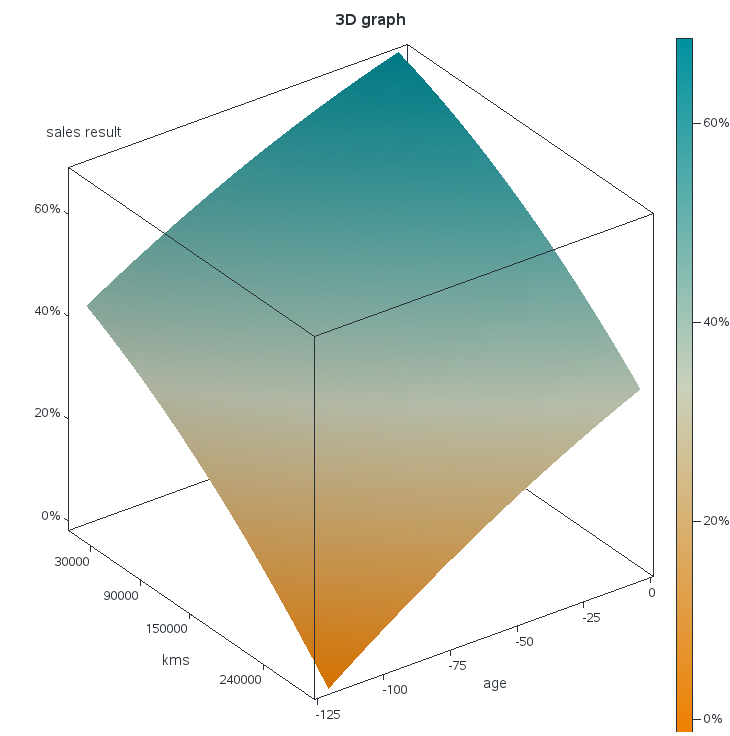## how to reverse the xaxis values

I use proc sgrender for a 3D surfaceplot.

Everything works fine except that the xaxis and yaxis do not meet at their highest values.

I do not find the option to reverse the axis. The x values are multiplied by -1 to achieve the wanted visualization, but the xaxisvalues now show negative values.

I wanted to trick the code by creating a format to show positive xaxisvalues again.

But it doesn't work.

For the context I attach my code, but you cannot use it like this. It should serve to put you in the picture.``````
proc template;                        /* surface plot with continuous color ramp */
define statgraph SurfaceTmplt;
dynamic _KMS _AGE _Z _Title;              /* dynamic variables */

begingraph;
entrytitle _Title;                   /* specify title at run time (optional) */
layout overlay3d / tilt=30
xaxisopts=(label="kms" linearopts=(tickvaluelist=(0 30000 60000 90000 120000 150000 180000 240000 300000 )) )
yaxisopts=(label="age" )
zaxisopts=(label="sales result" );

surfaceplotparm x=_KMS y=_AGE z=_Z /  /* specify variables at run time */

name="surface"
surfacetype=fill
colormodel=threecolorramp      /* or =twocolorramp */
colorresponse=_Z
reversecolormodel=true;
continuouslegend "surface";

endlayout;
endgraph;
end;
run;

data RISKNOBA.SCORED;
set RISKNOBA.SCORED;
_meses_efectivos=-meses_efectivos;
run;

proc format;
value abs low-high = [abs()];
run;

ods graphics / width=20cm height=20cm  ;
proc sgrender data=RISKNOBA.SCORED template=SurfaceTmplt;
where _RMCTMAIMG='6C122V' and byear=2021;
dynamic _KMS='_o_actual_mileage' _AGE='_meses_efectivos' _Z='P_it_based_sales_res' _Title="3D graph";
format P_it_based_sales_res percent7.0 _meses_efectivos abs.;
run;``````

2 REPLIES 2

## Re: how to reverse the xaxis values

Did you try placing the values in the TICKVALUELIST in reverse order?

## Re: how to reverse the xaxis values

Thanks @ballardw

Finally I've solved it using the negative value and using the tickvaluedisplaylist.

I'd like to use a format in the proc template, this would be smarter. But I don't know how.

``````proc template;                        /* surface plot with continuous color ramp */
define statgraph SurfaceTmplt;
dynamic _KMS _AGE _Z _Title;              /* dynamic variables */

begingraph;
entrytitle _Title;                   /* specify title at run time (optional) */
layout overlay3d / tilt=30
xaxisopts=(label="kms" linearopts=(tickvaluelist=(0 30000 60000 90000 120000 150000 180000 240000 300000 )) )
yaxisopts=(label="age" linearopts=(tickvaluelist=(-12 -24 -60 -84 -108 -120 )
tickdisplaylist=('12' '24' '60' '84' '108' '120')))
zaxisopts=(label="sales result" );

surfaceplotparm x=_KMS y=_AGE z=_Z /  /* specify variables at run time */

name="surface"
surfacetype=fill
colormodel=threecolorramp      /* or =twocolorramp */
colorresponse=_Z
reversecolormodel=true;
continuouslegend "surface";

endlayout;
endgraph;
end;
run;``````
Discussion stats
• 2 replies
• 151 views
• 0 likes
• 2 in conversation